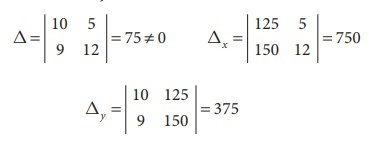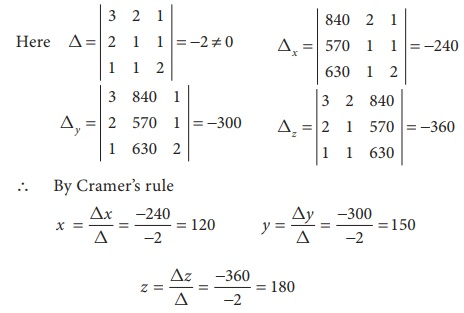Home | | Business Maths 12th Std | Cramer‚Äôs Rule

# Cramer‚Äôs Rule

i. Theorem (without proof) Cramer‚Äôs Rule, ii. Non Homogeneous linear equations upto three variables

Cramer‚Äôs Rule

Gabriel Cramer, a Swiss mathematician born in the city Geneva in 31 July 1704. He edited the works of the two elder Bernoulli‚Äôs, and wrote on the physical cause of the spheriodal shape of the planets and the motion of their apsides (1730), and on Newton‚Äôs treatment of cubic curves (1746).

In 1750 he published Cramer‚Äôs Rule, giving a general formula for the solution of certain linear system of n equations in n unknowns having a unique solution in terms of determinants. Advantages of Cramer‚Äôs rule is that we can find the value of x, y or z without knowing any of the other values of x, y or z. Cramer‚Äôs rule is applicable only when D ‚âÝ 0 ( is the determinant value of the coefficient matrix) for unique solution.

## Theorem (without proof) Cramer‚Äôs Rule:

Consider,If AX = B is a system of n linear equations in ‚Äòn‚Äô unknowns such that det (A) ‚âÝ 0, then the system has a unique solution.

This solution is,where Aj is the matrix obtained by replacing the entries in the jth column of A by the entries in the matrix## 1. Non Homogeneous linear equations upto three variables.

(a) Consider the system of two linear equations with two unknowns.The solution of unknown by Cramer‚Äôs rule is(b) Consider the system of three linear equations with three unknowns a1

x + b1 y + c1 z = d1

a2 x + b2 y + c 2 z = d2

a3 x + b3 y + c 3 z = d3Example 1.19

Solve the equations 2x + 3y = 7, 3x + 5y = 9 by Cramer‚Äôs rule.

Solution:

The equations are

2x + 3y = 7

3x + 5y = 9

Here‚à¥ we can apply Cramer‚Äôs Rule‚à¥ By Cramer‚Äôs rule‚à¥    Solution is      x = 8, y = ‚àí3

Example 1.20

The following table represents the number of shares of two companies A and B during the month of January and February and it also gives the amount in rupees invested by Ravi during these two months for the purchase of shares of two companies. Find the the price per share of A and B purchased during both the monthsSolution:

Let the price of one share of A be x

Let the price of one share of B be y

‚à¥ By given data, we get the following equations

10x + 5y = 125

9x + 12 y = 150‚à¥ By Cramer‚Äôs ruleThe price of the share A is ‚Çπ10 and the price of the share B is ‚Çπ5.

Example 1.21

The total cost of 11 pencils and 3 erasers is ‚Çπ 64 and the total cost of 8 pencils and 3 erasers is ‚Çπ49. Find the cost of each pencil and each eraser by Cramer‚Äôs rule.

Solution:

Let ‚Äòx‚Äô be the cost of a pencil

Let ‚Äòy‚Äô be the cost of an eraser

‚à¥ By given data, we get the following equations

11x + 3y =64

8x+3y=49‚à¥ The cost of a pencil is ‚Çπ 5 and the cost of an eraser is ‚Çπ 3.

Example 1.22

Solve by Cramer‚Äôs rule x + y + z = 4, 2x ‚àí y + 3z = 1, 3x + 2 y ‚àí z = 1

Solution:‚à¥ We can apply Cramer‚Äôs Rule and the system is consistent and it has unique solution.The solution is     ( x , y, z) = ( ‚àí1,3, 2)

Example 1.23

The price of 3 Business Mathematics books, 2 Accountancy books and one Commerce book is ‚Çπ840. The price of 2 Business Mathematics books, one Accountancy book and one Commerce book is ‚Çπ570. The price of one Business Mathematics book, one Accountancy book and 2 Commerce books is ‚Çπ630. Find the cost of each book by using Cramer‚Äôs rule.

Solution:

Let ‚Äòx‚Äô be the cost of a Business Mathematics book

Let ‚Äòy‚Äô be the cost of a Accountancy book.

Let ‚Äòz‚Äô be the cost of a Commerce book.

‚à¥ 3x + 2 y + z = 840

2x + y + z = 570

x + y + 2z = 630‚à¥ The cost of a Business Mathematics book is ‚Çπ120,

the cost of a Accountancy book is ‚Çπ150 and

the cost of a Commerce book is ‚Çπ180.

Example 1.24

An automobile company uses three types of Steel S1, S 2 and S3 for providing three different types of Cars C1, C2 and C3. Steel requirement R (in tonnes) for each type of car and total available steel of all the three types are summarized in the following table.Determine the number of Cars of each type which can be produced by Cramer‚Äôs rule.

Solution:

Let ‚Äòx‚Äô be the number of cars of type C1

Let ‚Äòy‚Äô be the number of cars of type C2

Let ‚Äòz‚Äô be the number of cars of type C3

3x + 2 y + 4z = 28

x + y + 2z = 13

2x + 2 y + z = 14‚à¥ The number of cars of each type which can be produced are 2, 3 and 4.

Tags : Theorem with Solved Example Problems , 12th Business Maths and Statistics : Chapter 1 : Applications of Matrices and Determinants
Study Material, Lecturing Notes, Assignment, Reference, Wiki description explanation, brief detail
12th Business Maths and Statistics : Chapter 1 : Applications of Matrices and Determinants : Cramer‚Äôs Rule | Theorem with Solved Example Problems